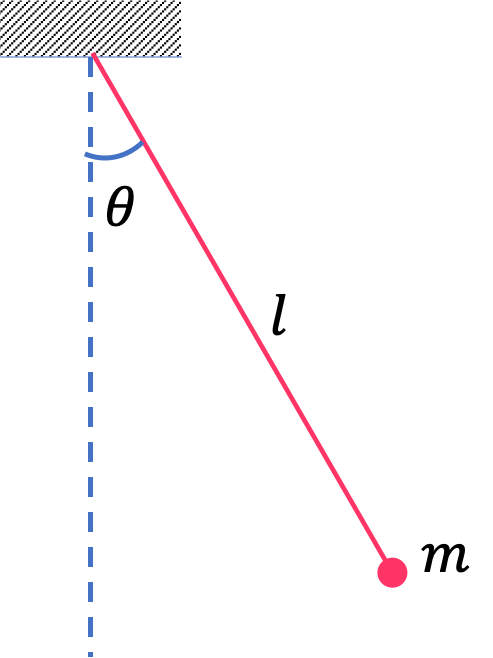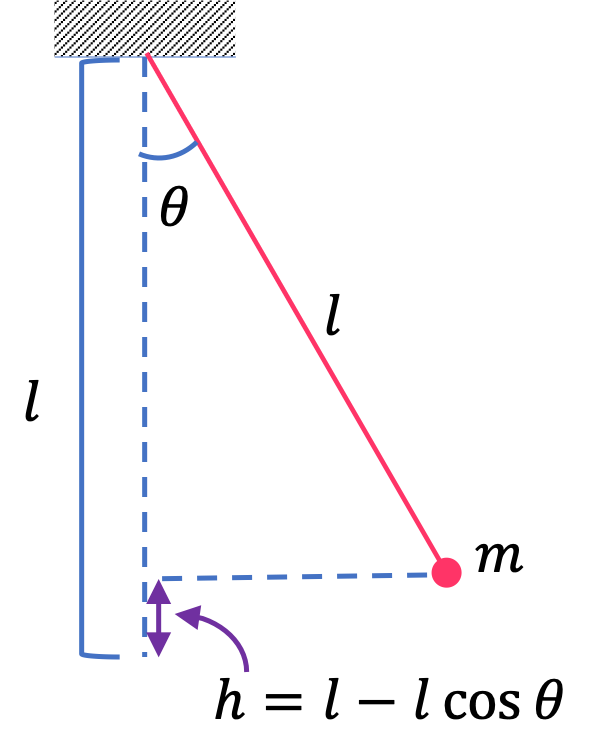## Section8.6Energy

The sum of kinetic and potential energies from all applicable conservative forces on an object is called its energy. We usually denote energy by the capital letter $E \text{.}$

\begin{equation} E = K + \sum U.\label{eq-energy-equal-to-K-plus-U}\tag{8.6.1} \end{equation}

Here $\sum$ sign indicates summation over all potential energies. For instance, if a block is attached to a spring and swings vertically, $\sum U$ will be $U_\text{weight} + U_\text{spring}\text{.}$

### Subsection8.6.1Energy of a Particle Subject to Gravity

For instance, if our system is a particle of mass $m$ moving with speed $v$ at some instant $t \text{,}$ and is subject to only the force of gravity. Then, with positive $y$ axis pointed up, its potential energy will be

\begin{equation*} U = mgy. \end{equation*}

Therefore, the energy of this particle at this instant will be

\begin{equation*} E = \dfrac{1}{2}mv^2 + mgy. \end{equation*}

### Subsection8.6.2Energy of a Particle Subject to Gravity and Spring Forces

Now, suppose, the aforementioned particle was attached to a spring of spring constnat $k \text{.}$ Let the spring at instant $t$ was either compressed or stretched by $\Delta l\text{.}$ Then, the potential energy would be a sum of the potential energies due to gravity and due to the spring force.

\begin{equation*} U = mgy + \dfrac{1}{2}k \left( \Delta l\right)^2. \end{equation*}Therefore, energy of this particle will be

\begin{equation*} E = \dfrac{1}{2}mv^2 +mgy + \dfrac{1}{2}k \left( \Delta l\right)^2. \end{equation*}
###### Remark8.6.1.Definition of Energy as a Guide.

The definition of energy, as sum of kinetic and potential energies, given in Eq. (8.6.1) is a guide for you to find the actual expression in your particular situation. In each situation, you would need to ask, what are the conservative forces, and set up coordinates or variables that are necessary for properly expressing protential energy.

A car of mass $2,000 \text{ kg}$ is moving up a hill at a speed of $25 \text{ m/s}$ as shown in Figure 8.6.3. The angle of inclination of the hill is $10^{\circ}\text{.}$ If the bottom of the hill is chosen to be the zero of gravitational potential energy, what is the energy of the car when it is at a location that is $150\text{ m}\text{,}$ as measured on the incline, from the bottom of the hill?

Hint

Find the height above the bottom and use that calculate the potential energy.

$1,136,000\text{ J}\text{.}$

Solution

The energy will be the sum of kinetic and potential energies. For potential energy due to gravity, we need height from the zero reference. Here $h = 150\text{ m} \times \sin\,10^{\circ} = 26.05\text{ m}.$ Therefore, energy is

\begin{align*} E \amp = \dfrac{1}{2}mv^2 + m g h, \\ \amp = \dfrac{1}{2}\times 2,000\times 25^2 + 2,000\times 9.81\times 26.05, \\ \amp = 625,000+ 511,000 = 1,136,000\text{ J}, \end{align*}

where I have rounded to four significant figures.

A plane pendulum of length $l$ is oscillating about its equilibrium. Let $\theta$ be the angular displacement from vertical at an arbitrary instant. The pendulum bob has mass $m$ and has speed $v$ at this instant. What is the energy of the pendulum bob?Hint

The height from equilibrium is $h = l - l \, \cos\, \theta \text{.}$

$\dfrac{1}{2}m v^2 + m g \left( l - l \, \cos\, \theta \right)\text{.}$

Solution

The figure here shows height $h$ of the bob from the zero potential energy reference, which happens when the pendulum is vertical. From the adjacent side of the triangle and the length of the string, we get

\begin{equation*} h = l - l \, \cos\, \theta. \end{equation*}The gravitational potential energy will be $m g h\text{.}$ The energy of the pendulum bob will be

\begin{equation*} E = \dfrac{1}{2}m v^2 + m g \left( l - l \, \cos\, \theta \right). \end{equation*}

A block of mass $m$ is attached to two springs of spring constants $k_1$ and $k_2$ as shown in Figure 8.6.6. When the block is in the middle, the two springs are neither stretched nor compressed.

When block is pulled to one side, one of the spring is compressed while the other is stretched by the same amount. At some point in time, the block has a speed $v$ and at that instant the spring with spring constant $k_1$ is stretched by an amount $d\text{.}$ Assume the block moves on a horizontal frictionless table.

What is the energy of the block at this instant?

Hint

Add two PEs to one KE.

$\dfrac{1}{2} m v^2 + \dfrac{1}{2} k_1 d^2 + \dfrac{1}{2} k_2 d^2$ .

Solution

We add kinetic energy to the two potential energy terms, from the two conservative forces by the two springs.

\begin{equation*} E = \dfrac{1}{2} m v^2 + \dfrac{1}{2} k_1 d^2 + \dfrac{1}{2} k_2 d^2. \end{equation*}

The potential energy due to gravitational force between two bodies is given by the formula:

\begin{equation*} U = - G\dfrac{m_1m_2}{r}. \end{equation*}

At some instant Moon is a distance $r_{em}$ from the Earth and a distance $r_{sm}$ from the Sun. At this instant the speed of Moon is $v_m\text{.}$ Let $M_m,\ M_e,\ M_s$ be the masses of the Moon, the Earth, and the Sun. What is the energy of Moon?

Hint

Add two PEs to one KE.

$\dfrac{1}{2}\,M_m\, v_m^2$ $-G\, M_m \left( \dfrac{M_e}{r_{em}} + \dfrac{M_s}{r_{sm}} \right)$ .